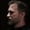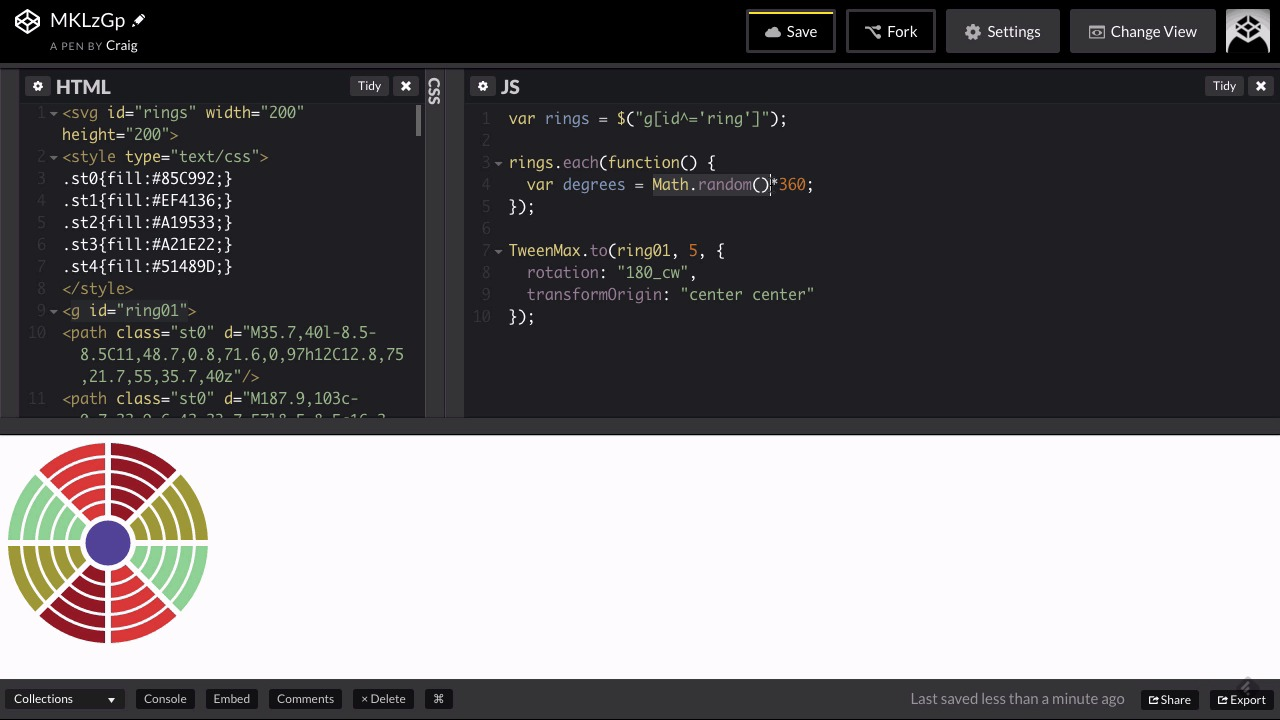Lessons: 18Length: 2.3 hours• Overview
• Transcript

# 7.2 Random Rotation

In this lesson, you will set up random rotations for each of the rings in your SVG object using the DirectionalRotationPlugin.

## 8.Conclusion1 lesson, 00:42

### 7.2 Random Rotation

Hello and welcome back to Advanced Animation with GSAP plugins. In this lesson we're going to continue our discussion of the directional rotation plugin. And we're gonna pick up where we left off in the last lesson and the starting code pin that we're working with here, can be found in your course notes. So once you open that up, go ahead and click on Fork to create a new copy of it. And then we'll start with our new copy. So I've collapsed the CSS section here. And right now all we're doing is pointing to a single ring and we've set a starting rotation for it of 45 degrees. And then we've created a tween, where it's animating to a value of 180 degrees moving in the clockwise direction. I'm gonna get rid of the starting value here where we set the initial value. And what I want to do now is I want to randomize the rotation of each of these rings. Now we have five separate rings and each one of them has a different id. So we have one with an id of ring01, we have ring02, 03 etc. So I want to store all of these rings inside one variable. So we're gonna call this variable rings instead of ring01 and our selector here is going to get a little more complex because I want to grab everything that has an ID that starts with a ring. So we're gonna have again, a complex selector here and the way we do this is inside square brackets. Notice that we're still inside quotation marks here, that's always needed. But inside square brackets here, I'm gonna point to the id attribute and I want the id to start with ring, it can have 01 after it that's fine but we want to start with ring. And we also want to make sure that it's a group, not an SVG because you'll notice the SVG itself has an id that starts with ring as well and we don't want to apply it to this. We want to apply it to each of the groups inside that SVG. So just to the left of that opening square bracket, I'm gonna put a g. Notice there's no space here if you put a space here that will mess things up. So g and then inside square brackets we're gonna have id and then instead of equals ring01 or something like that, we wanted to start with a value of ring. So what we're gonna do is we're gonna say id ^= ring inside single quotes. So this ^= and that caret right there is on your 6 key on your keyboard. So if you hit Shift+6 that will give you the caret character. If you have ^= there that means that your id is going to start with whatever is inside the single quotes here. So we're looking at any group that has an id that starts with ring. So that should get us our entire array of rings. So each one of these groups will be stored inside this variable. So now we can create a function for each one of these individually by pointing to that rings variable and then saying .each and this is a jQuery thing that we're doing here. So if we say .each and then inside parentheses we type function, and then will nudge our closing curly bracket and parentheses down a couple lines and put a semicolon at the end. And then inside that function we can create some code here that's going to run for each one of these individual rings. So what I want to do for each ring is to create a random number that we're going to animate to, and this number is going to be in degrees. So we'll create a variable here that I'm gonna call degrees. And we're going to set it equal to Math.random, lowercase r, open and close parentheses. And then we're gonna multiply that by 360. So let me explain what this is doing, and we're gonna add more to this in just a second. But Math.random will basically give us a random number between 0 and 1. So it could be 0, it could be 1, it could be 0.3256. And if we multiply that value by 360, then that will give us a random number between 0 and 360. So any time you want to random number between 0 and another number, you simply multiply your Math.random by that higher number and that should give you a random number again between 0 and 360. Now this is gonna give us some kind of crazy number, so I want to round it off just to make it a little bit easier. So we're gonna round it off and make it a whole number. So the way we round something is we would say something like this Math.round and then inside parentheses we might say something like 4.5 and it would round it up to 5. Well, instead of 4.5, inside this parentheses here for Math.round we're gonna put this entire equation here Math.random()*360. So I'm gonna cut that, we'll get rid of the extra semicolon there and then inside the Math.round function we're gonna paste that. And that should get us our number in degrees that we're gonna use. And so for each ring that runs this function, it's gonna come up with a different random number. So now we can create a tween that animates from wherever its starting position is, which is by default a rotation of 0 to whatever this random number is that we came up with. So we're gonna create our tween here. So TweenMax.to, and then we're going to be animating the whatever particular ring we're currently looking at. So, we're gonna look at the current ring and the way we do that is we're going to use this jQuery notation here again. So we have a $() and inside the parentheses we're gonna point to this. Notice that that's not inside quotation marks. So we just have$(this) that will point to whichever current ring we're talking about. So when we were pointing to rings.each, whichever current ring is being evaluated at the time this function runs that's going to be indicated by this \$(this). So we're gonna animate this current item and let's say we want it to take ten seconds so it's going to be a nice slow animation. And then we're gonna have our opening and closing curly brackets for that animation. So for this animation we're going to rotate it. So we'll set our rotation property to a value of degrees, which is gonna be this random number that we came up with. But then we're also going to be using our directional rotation plugin to, in this instance, move them all in a clockwise direction. So we need to _cw, not cow [LAUGH]. Sorry, cw. So we have the number of degrees and then we're going to append to the end of that _cw to make sure that it's all moving clockwise. Now as you can see over here, our transformation origin needs to be adjusted because it's set in the upper left hand corner. So after our rotation property here we're going to type comma, go to the next line, and we're going to set the transform origin property. And we're gonna set that to a value of center center, so that will align it to the horizontal and vertical centers, and now we can see that animating appropriately. So everything is animating to its own random number and at the end of ten seconds we can see where it ends up. And that's how you can randomize the rotation of your objects, again, using this directional rotation plug. And so right now we are assuming that we want to move everything in a clockwise direction. If you wanted to you could go counterclockwise or short, whatever you prefer, but for now I'll leave it at clockwise. So again, that's how you randomized your rotations in GreenSock. Thank you for watching and we'll see you in the next lesson.

Back to the top